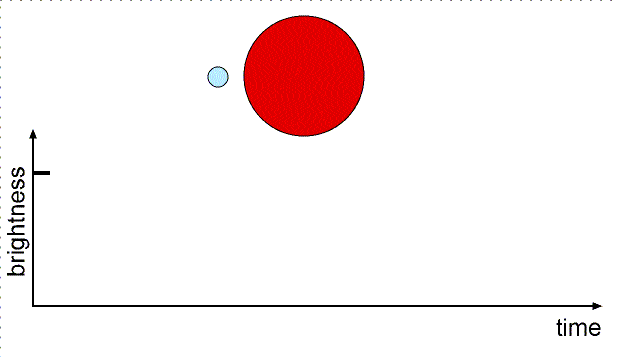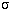### The Sizes of Stars

#### Chapter index in this window —   — Chapter index in separate window

This material (including images) is copyrighted!. See my copyright notice for fair use practices.

All but a few stars appear as mere pinpoints in even the largest telescopes. They are much too far away to derive their diameters from measuring their angular diameters and distances. Eclipsing binaries are used to determine indirectly the diameters of stars. These are two stars orbiting each other in a plane that is parallel to your line of sight so you see their orbits edge-on. This means that one star will periodically cover up the other star. During the eclipse the total brightness measured for the binary will decrease. The amount of the dip in brightness depends on the luminosity and relative size of the two stars.

A star's diameter is found from speed = (distance travelled)/(time it takes). The speed comes from the doppler shift and the time is the length of the eclipse. The distance travelled during the eclipse is equal to the diameter of the star = 2 × radius. The light curve---plot of brightness vs. time---is used to derive the star diameters. Here is an example of two stars orbiting each other in circular orbits seen edge-on with one star small and hot and the other large and cool:When the small star moves from position 1 to position 2 (or from position 3 to position 4), it has moved a distance equal to its diameter. When the small star moves from position 1 to position 3 (or from position 2 to position 4), it has moved a distance equal to the diameter of the large star.

Star sizes can also be found (less accurately) from the luminosity and the flux. Recall from the magnitude section above that the luminosity = [4p×(star radius)2] × [×(star's surface temperature)4], whereis the Stefan-Boltzmann constant. If you compare the star with the Sun, you can cancel out the constants to get (star's radius)/(Sun's radius) = (Sun's temperature/star's temperature)2 × Sqrt[star's luminosity/Sun's luminosity]. See the ``How do you do that?'' box below for an example. The sizes of different types of stars are summarized in the Main Sequence Star Properties table below.

### How do you do that?

Antares is 9120 times more luminous than the Sun (Antares' luminosity/Sun's luminosity)= 9120) and has a temperature of only 3340 K and the Sun's temperature is 5840 K.

Antares' size/Sun's size = (5840/3340)2 × Sqrt = 3.057 × 95.5 = 292. Antares is almost 300 times the size of the Sun! If the Sun were replaced by Antares, the inner planets Mercury, Venus, and Earth would be inside Antares! It is a red giant star---a star close to death.

Try other scenarios of the star sizes and star masses with the UNL Astronomy Education program's Eclipsing Binary Simulator (link will appear in a new window). You can adjust the star masses, sizes, temperatures and separations and orbit inclination and eccentricity to see how the light curve changes.

#### Vocabulary

light curve eclipsing binary

#### Formulae

• Eclipsing binary: diameter = speed × time of eclipse.
• Size from luminosity: star's radius/Sun's radius = (Sun's temperature/star's temperature)2 Sqrt[star's luminosity/Sun's luminosity].

#### Review Questions

1. How do you use the light curve to find the diameters of stars?
2. What special type of binary star system is used to find the diameters of stars?
3. Use the light curve in the figure in the section above. Assume that when star A is behind star B, the small dip in brightness is seen. When star B is behind star A, the big dip in brightness is seen. Which star is more luminous?
4. From the previous problem, if t1 = 45 minutes, t2 = 60 minutes, t3 = 105 minutes, t4 = 120 minutes, what is (star A diameter)/(star B diameter)? [Hint: find which star is brighter and in this circular orbit system (t8 - t6) = (t4 - t2).]
5. From the previous problem, if the velocity is 750 kilometers/second, what is the diameter of the larger star?
6. The white dwarf Sirius B has a temperature of 14,000 K and a luminosity only 0.00794 times the Sun's luminosity. What is the diameter of Sirius B in kilometers? (The Sun's radius = 696,000 kilometers.)

#### Go to Astronomy Notes home

last updated: August 20, 2007